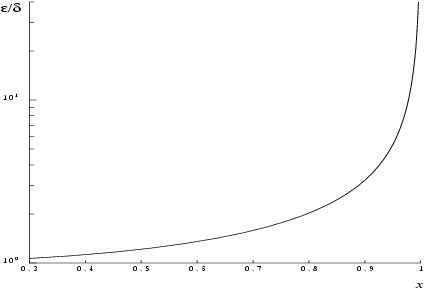NAG Library Function Document

1Purpose

nag_arctanh (s11aac) returns the value of the inverse hyperbolic tangent, $\mathrm{arctanh}x$.

2Specification

 #include #include
 double nag_arctanh (double x, NagError *fail)

3Description

nag_arctanh (s11aac) calculates an approximate value for the inverse hyperbolic tangent of its argument, $\mathrm{arctanh}x$.
For ${x}^{2}\le \frac{1}{2}$ it is based on the Chebyshev expansion
 $arctanh⁡x=x×yt=x∑′r=0arTrt$
where $-\frac{1}{\sqrt{2}}\le x\le \frac{1}{\sqrt{2}}$, $\text{ }-1\le t\le 1$, $\text{ and }t=4{x}^{2}-1$.
For $\frac{1}{2}<{x}^{2}<1$, it uses
 $arctanh⁡x=12ln1+x 1-x .$
For $\left|x\right|\ge 1$, the function fails as $\mathrm{arctanh}x$ is undefined.
Abramowitz M and Stegun I A (1972) Handbook of Mathematical Functions (3rd Edition) Dover Publications

5Arguments

1:    $\mathbf{x}$doubleInput
On entry: the argument $x$ of the function.
Constraint: $\left|{\mathbf{x}}\right|<1.0$.
2:    $\mathbf{fail}$NagError *Input/Output
The NAG error argument (see Section 3.7 in How to Use the NAG Library and its Documentation).

6Error Indicators and Warnings

NE_ALLOC_FAIL
Dynamic memory allocation failed.
See Section 2.3.1.2 in How to Use the NAG Library and its Documentation for further information.
NE_INTERNAL_ERROR
An internal error has occurred in this function. Check the function call and any array sizes. If the call is correct then please contact NAG for assistance.
See Section 2.7.6 in How to Use the NAG Library and its Documentation for further information.
NE_NO_LICENCE
Your licence key may have expired or may not have been installed correctly.
See Section 2.7.5 in How to Use the NAG Library and its Documentation for further information.
NE_REAL_ARG_GE
On entry, ${\mathbf{x}}=〈\mathit{\text{value}}〉$.
Constraint: $\left|{\mathbf{x}}\right|<1$.
The function has been called with an argument greater than or equal to $1.0$ in magnitude, for which $\mathrm{arctanh}$ is not defined.

7Accuracy

If $\delta$ and $\epsilon$ are the relative errors in the argument and result, respectively, then in principle
 $ε≃ x 1-x2 arctanh⁡x ×δ .$
That is, the relative error in the argument, $x$, is amplified by at least a factor $\frac{x}{\left(1-{x}^{2}\right)\mathrm{arctanh}x}$ in the result. The equality should hold if $\delta$ is greater than the machine precision ($\delta$ due to data errors etc.) but if $\delta$ is simply due to round-off in the machine representation then it is possible that an extra figure may be lost in internal calculation round-off.
The behaviour of the amplification factor is shown in the following graph:Figure 1
The factor is not significantly greater than one except for arguments close to $\left|x\right|=1$. However in the region where $\left|x\right|$ is close to one, $1-\left|x\right|\sim \delta$, the above analysis is inapplicable since $x$ is bounded by definition, $\left|x\right|<1$. In this region where arctanh is tending to infinity we have
 $ε∼1/ln⁡δ$
which implies an obvious, unavoidable serious loss of accuracy near $\left|x\right|\sim 1$, e.g., if $x$ and $1$ agree to $6$ significant figures, the result for $\mathrm{arctanh}x$ would be correct to at most about one figure.

8Parallelism and Performance

nag_arctanh (s11aac) is not threaded in any implementation.

None.

10Example

This example reads values of the argument $x$ from a file, evaluates the function at each value of $x$ and prints the results.

10.1Program Text

Program Text (s11aace.c)

10.2Program Data

Program Data (s11aace.d)

10.3Program Results

Program Results (s11aace.r)

© The Numerical Algorithms Group Ltd, Oxford, UK. 2017